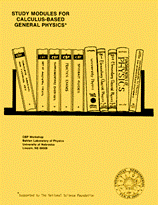Instructional Materials in Physics and AstronomyCalculus-Based General Physics

Title

Rotational Motion

Date of this Version

1975

From Study Modules for Calculus-Based General Physics
Reproduction rights granted.

Abstract

There is a motion of a system of masses that is as simple as the motion of a point mass on a straight line. It is the rotation of a rigid body about a fixed axis. For example, we live on a rotating earth, use rotating devices such as a potter's wheel or a phonograph turntable, and test our luck with a spinning roulette wheel. All of these are objects whose motion is described by the time dependence of a single variable, the angle of rotation. We shall study the angular equivalent of uniformly accelerated motion for some rotating objects. This module also begins the study of rotational dynamics by introducing the dynamical quantities torque and angular momentum for a point mass moving in a plane.

COinS